Function Repository Resource:

# BooleanCompose

Functionally compose a Boolean expression

Contributed by: Jesse Friedman
 ResourceFunction["BooleanCompose"][expr] represents a composition of the variables in the Boolean expression expr, where each variable is a function.

## Details and Options

ResourceFunction["BooleanCompose"] allows you to “pre-compose” logic which can later be applied to a value.
Each of the Boolean variables in expr is treated as a function and is applied to the argument or arguments the composition as a whole is applied to, after which the results are substituted in place of their corresponding variables and the Boolean expression is evaluated.
The expression expr can involve any combination of Boolean functions supported by BooleanVariables, such as And, Or, Not, and arbitrary BooleanFunction objects.

## Examples

### Basic Examples (1)

Create a predicate that returns True if its argument is both positive and an even number:

 In:=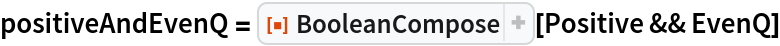Out=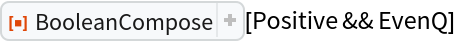In:=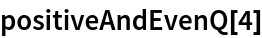Out=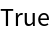In:=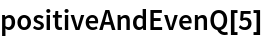Out=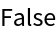In:=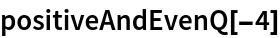Out=### Scope (6)

Pure functions can be used in the composition:

 In:=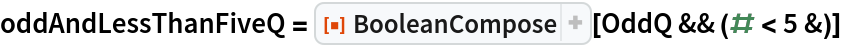Out=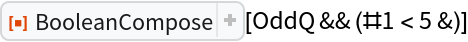In:=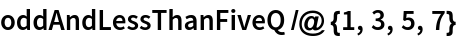Out=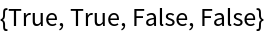The composition can be applied to multiple arguments:

 In:=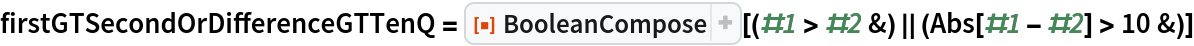Out=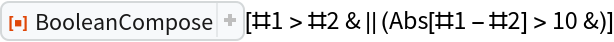In:=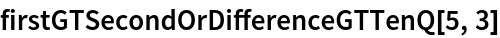Out=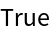In:=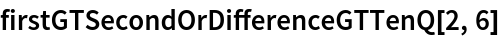Out=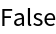In:=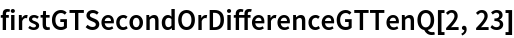Out=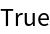Multiple identical Boolean variables are only applied once each:

 In:=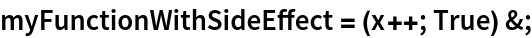In:=Out=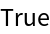In:=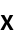Out=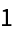If any of the Boolean variables are not functions, applying the composition may yield a symbolic result:

 In:=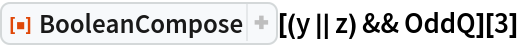Out=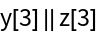However, if the Boolean expression can be short-circuited, a Boolean value will be returned:

 In:=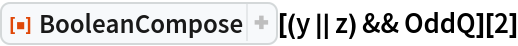Out=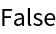All of the basic Boolean operators are supported:

 In:=Out=In:=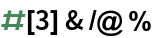Out=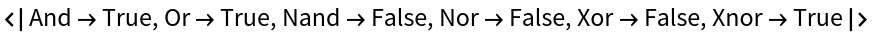BooleanFunction is supported:

 In:=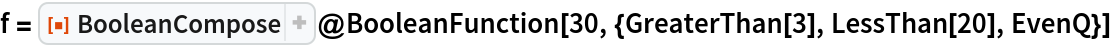Out=In:=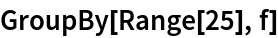Out=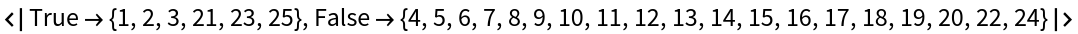### Applications (2)

Improve code readability by using functional-style composition:

 In:=In:=Out=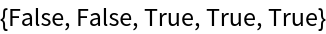Compare to a more conventional pure function-based approach:

 In:=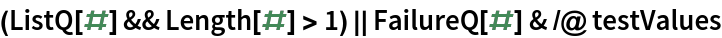Out=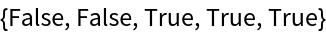While slightly more verbose, some may prefer the literacy and reduced duplication of the compositional style.

### Possible Issues (2)

Every unique function in the Boolean expression will be applied to the argument(s) that the composition is applied to, even if the expression can be short-circuited:

 In:=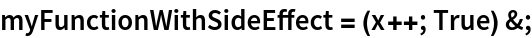In:=Out=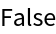In:=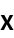Out=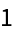The exception to this is the case where the expression is automatically simplified by the parser due to presence of a raw Boolean value:

 In:=Out=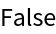In:=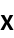Out=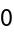Jesse Friedman

## Version History

• 2.0.0 – 29 April 2020
• 1.0.0 – 22 October 2019